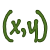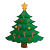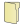Games
Problems
Go Pro!

# Systems of Equations

Pro Problems > Math > Algebra > Equations > Systems of Equations

# Featured Pro ProblemsFind the sum of x and y, if the following quadratic equations in x and y are true.Two people go to the same store and make the same purchases, but one of them is treated unfairly in the pricingThe sum of two numbers is six more than twice the smaller number, and if the larger number is multiplied by three, the result is ten less than seven...A hypothetical problem in which the treasury introduces a new coin into circulationDetermine how many floors a skyscraper has, based on information about someone climbing the stairsWhat happened after Humpty Dumpty had his shattering fallHermie the Elf goes Christmas shopping, but all he has is coins in his pocketSolve the system of equations which involves a product of variables# Full Directory Listing

b Over X + Y, Find X + Y + Z, Fractions within Fractions, P and Q, P and Q, System in a System, System of Equations with K, System of Three Equations, Three Numbers, Three to Two, Ugly Fractional SystemLike us on Facebook to get updates about new resources